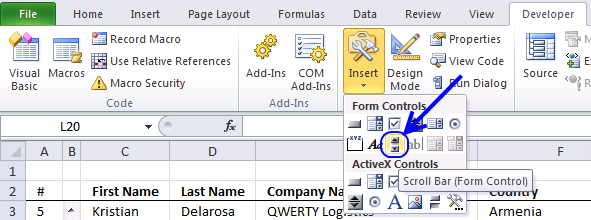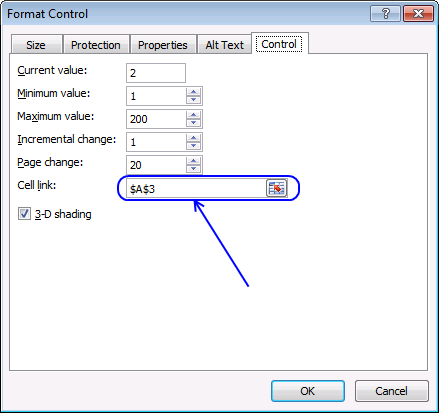Author: Oscar Cronquist Article last updated on October 17, 2018

Sometimes you just want to show a small section of your data set, like in a dashboard or a chart. With a scroll bar or spin buttons you can accomplish that easily.

Clicking the up or down arrows on the scroll bar changes the current record in cell range C3:F3. Below the cell range is the excel table where all the data is extracted from.

### Here is how I did it

Insert a scroll bar

1. Go to tab Developer on the ribbon
2. Click "Insert" button3. Click Scroll bar
4. Drag on sheet to create a scroll bar
5. Right click on scroll bar
6. Click "Format control"7. Change cell link to \$A\$3
8. Click OK

Enter formulas

Array formula in cell C3:F3:

=INDEX(Table1,A3,0)
1. Select cell range C3:F3
2. Type =INDEX(Table15,A3,0)
3. Press and hold CTRL + SHIFT
4. Press Enter

Use an event (vba) to automatically change scroll bar maximum range value

Activating the sheet runs the macro and sets the maximum value for the scroll bar. Example, if you add values to the table and it now has 250 rows. The event macro changes the scroll bar maximum range value to 250.

```Private Sub Worksheet_Activate()
With ActiveSheet.Shapes("Scroll Bar 1").ControlFormat
.Min = 1
.Max = Range("Table1").Rows.Count
.SmallChange = 1
.LargeChange = Range("Table1").Rows.Count / 10
.LinkedCell = "\$A\$3"
End With
End Sub

```
1. Right click on sheet name
2. Click on "View Code"
3. Paste vba code to module
4. Exit VB Editor and return to excel

The following example demonstrates scrolling through 10 records at a time. Clicking the scroll bar arrows changes the values in cell range C2:F12.

Formula in cell C3:

=IFERROR(INDEX(Table2,\$A\$3+ROW(A1)-1,COLUMN((A1))),"")

Copy cell C3 and paste to cell range C3:F12.

Formula in cell A4:

=A3+1

Copy cell A4 and paste to cell range A5:A12

This example shows a scrolling excel chart.

The last example shows conditional formatting highlighting current record.

Conditional formatting formula

=(\$C8=\$C\$3)*(\$D8=\$D\$3)*(\$E8=\$E\$3)*(\$F8=\$F\$3)

### Download excel *.xlsm file

Scroll through records.xlsm

### Functions in this post

INDEX(array, row_num, [column_num])

Returns a value or reference of the cell at the intersection of a particular row and column, in a given range.